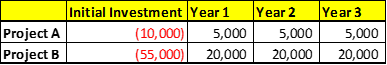# Using the Payback Method, IRR, and NPV

The purpose of this assignment is to allow the student to calculate the project cash flow using net present value (NPV), internal rate of return (IRR), and the payback methods.

Assignment Steps

Resources: Corporate Finance

Create a 350-word memo to management including the following:

• Describe the use of internal rate of return (IRR), net present value (NPV), and the payback method in evaluating project cash flows.

Calculate the following time value of money problems:

1. If you want to accumulate \$500,000 in 20 years, how much do you need to deposit today that pays an interest rate of 15%?
2. What is the future value if you plan to invest \$200,000 for 5 years and the interest rate is 5%?
3. What is the interest rate for an initial investment of \$100,000 to grow to \$300,000 in 10 years?
4. If your company purchases an annuity that will pay \$50,000/year for 10 years at a 11% discount rate, what is the value of the annuity on the purchase date if the first annuity payment is made on the date of purchase?
5. What is the rate of return required to accumulate \$400,000 if you invest \$10,000 per year for 20 years. Assume all payments are made at the end of the period.

Calculate the project cash flow generated for Project A and Project B using the NPV method.

• Which project would you select, and why?
• Which project would you select under the payback method? The discount rate is 10% for both projects.